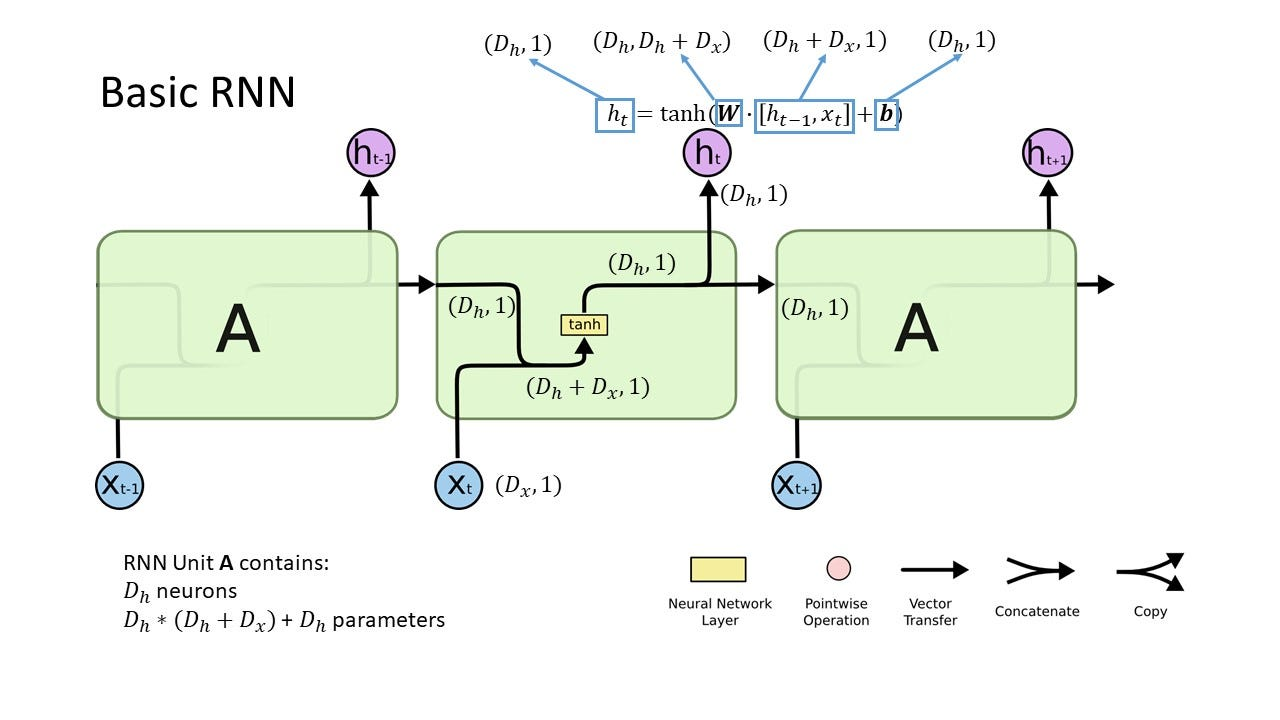# Understand Recurrent Neural Network with Four Figures

Recurrent neural network is gaining popularity in sequence modeling such as natural language processing and time series forecasting. Sometimes, it is not as straight forward as convolutional neural network and difficult to fully understand. Through reading blogs and taking coursera courses (deep learning specialization), I’m coming up with four figures hopefully helpful for you to understand RNN better. The figures in this blog is built upon the figures from Colah’s blog: http://colah.github.io/posts/2015-08-Understanding-LSTMs/

The goal of this blog is to answer four main questions in RNN:

1. What is a RNN unit/cell?
2. What is called a gate?
3. How data dimensions are transformed within RNN unit?
4. What is the difference between LSTM and GRU?

What is a RNN unit/cell

A RNN unit/cell consists of several single neural network layers. It is the basic operating unit in RNN. A simple RNN unit can be as simple as one layer of neurons, as shown in Fig 1. It takes the input and the output from the previous unit, concatenates them together (dimensions will be the sum of two dimensions) as the input to the neural layer, and output via an activation function (tanh). The neural network layer transforms the input dimension (D_h+D_X) to the output dimension D_h. The number of neurons and the number of weights are shown in Fig 1, along with the dimension transformation. In the notation, we put the number of dimensions first and the number of samples second. (D_x, 1) means 1 sample with D_x dimensions.Fig 1. Basic RNN: RNN unit and data transformation within the unit.

What is a gate

A gate controls whether or not to pass on the information. Mathematically, it is basically 0 or 1. 0 means closing the gate and 1 means opening the gate with all information passing on. A sigmoid layer is perfect for representing 0 and 1. The output of sigmoid is a value varying between 0 and 1. The output controls the degree of opening the gate. The closer to 1, the gate is more open. Then we multiply the output from the sigmoid layer with the information we want to open or close. Therefore, a gate consists of two components: a sigmoid neural network layer and a multiplication operator.

Let’s take LSTM (long short term memory) as an example. We can easily identify all the gates by looking for sigmoid layer and multiplication operators. Then we can identify the function of the gate by looking for what information this multiplication operator is applied to. If the operator is on cell state from prior unit, it is forget gate, indicating how much prior information to carry over. If the operator is on the input X, it is the input gate. If the operator is before the final output, it is the output gate. This way, we can dissect the LSTM structure fairly easily. You may wonder what is the function of the tanh layer in the LSTM unit. Since the input X can be any value, we want to map the value to a controlled range and also add some non-linearity. After the sum of prior information and input, we use a tanh operator (not a layer, so no dimension transformation here!) to scale the output to [-1,1]. We always want to keep our input and output values to a known range to avoid too large numbers. The gates are highlighted in Fig. 2 and the corresponding dimension transformation is highlighed in Fig. 3.

Comparison of LSTM and GRU

LSTM was proposed in 1997, while GRU was proposed recently in 2014. They are two most popular RNN units used nowadays. We can see that the design of a RNN unit is basically a design of gate: how can we find the cell state and the output from the current input and prior cell state. Now we know the gate consists of a sigmoid layer and a multiplication operator, we need to consider what information we want to consider for the gate input, and what information we want to operate the gate on.

Now we can easily identify the gates in the RNN unit. We can see that in LSTM, all gates are seperate, controlled by seperate sigmoid layers. In GRU, forget gate and input gate is merged together. For the forget gate, there is a “1-” operator. If the sigmoid output is 0.4, it means 0.4 information (input and the prior cell state) will be kept and 0.6 information (prior cell state) will be forgotten. In GRU, there is no output gate, since the cell state and output are the same. There is an additional gate that LSTM does not have: relevant gate. It operates on the prior cell state before concatenating the input. The relevant gate tells how relevant the previous cells state is to current cell state. We can see that GRU has only three neural network layers while LSTM has four layers. GRU introduces 3/4 of the parameters of LSTM, which will require less time to train.